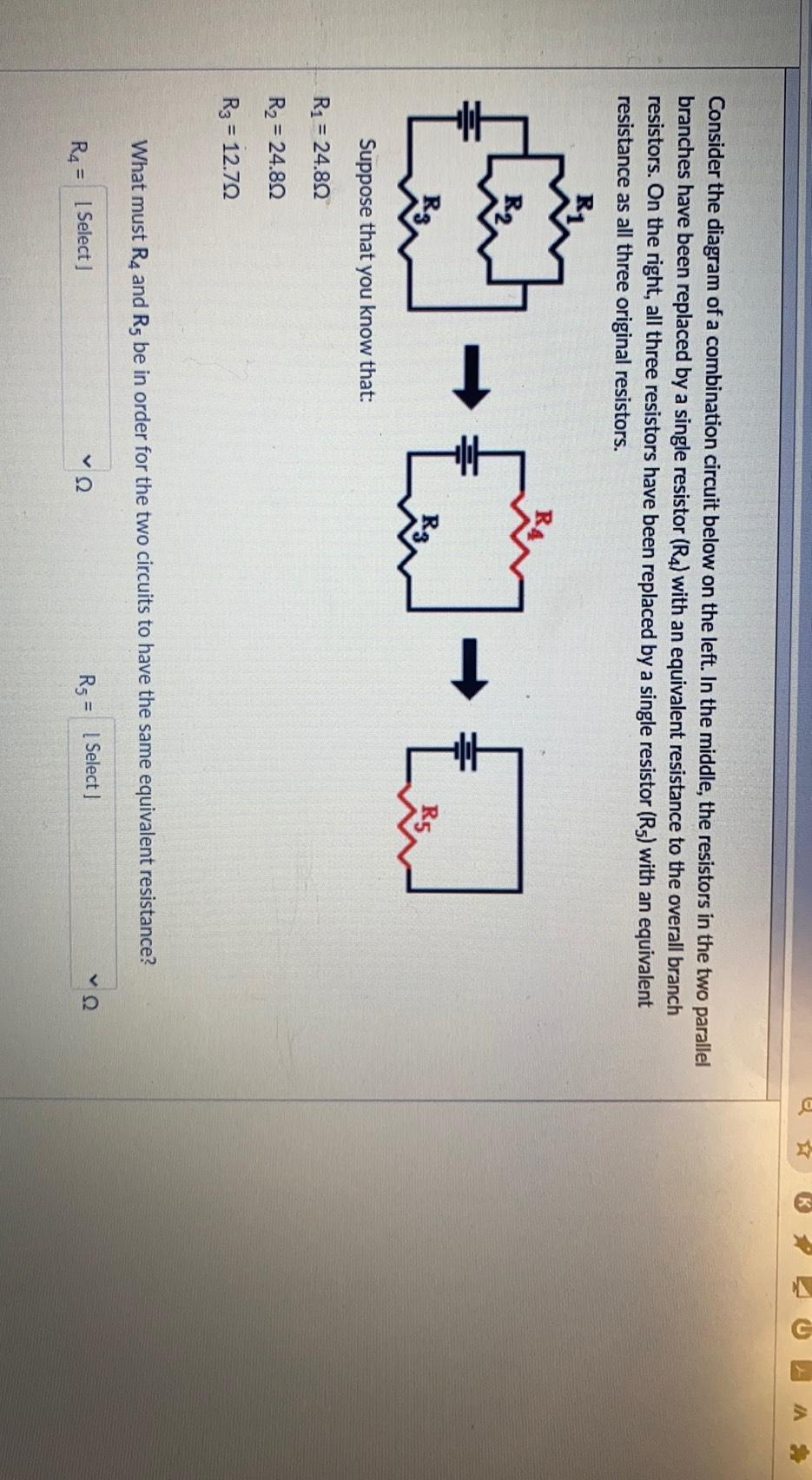Question:

# * K Consider the diagram of a combination circuit below on the left. In the middle, the resistors in the two parallel branches h* K Consider the diagram of a combination circuit below on the left. In the middle, the resistors in the two parallel branches have been replaced by a single resistor (R4) with an equivalent resistance to the overall branch resistors. On the right, all three resistors have been replaced by a single resistor (R5) with an equivalent resistance as all three original resistors. thew - £ Rz R5 Suppose that you know that: R2 = 24.80 R2 = 24.892 R3 = = 12.722 What must R4 and Rs be in order for the two circuits to have the same equivalent resistance? R4 = [ Select] v2 V22 Rs = [Select]